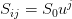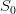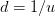# A New Kind of Binomial Tree

We can port even more complicated problems from mathematics directly to a functional language. For an example closer to home, let us consider a binomial pricing model, illustrating that the ease and elegance with which Haskell handles factorial do indeed extend to real-life quantitative finance problems as well.

The binomial tree pricing model works by assuming that the price of an underlying asset can only move up or down by constant factors$u$ and$d$ during a small time interval$\delta t$. Stringing together many such time intervals, we make up the expiration time of the derivative instrument we are trying to price. The derivative defined as a function of the price of the underlying at any point in time.Figure 1. Binomial tree pricing model. On the X axis, labeled i, we have the time steps. The Y axis represents the price of the underlying, labeled j. The only difference from the standard binomial tree is that we have let j be both positive and negative, which is mathematically natural, and hence simplifies the notation in a functional language.

We can visualize the binomial tree as shown in Fig. 1. At time$t = 0$, we have the asset price$S(0) = S_0$. At$t = \delta t$ (with the maturity$T = N\delta t$). we have two possible asset values$S_0 u$ and$S_0 d = S_0 / u$, where we have chosen$d = 1/u$. In general, at time$i\delta t$, at the asset price node level$j$, we have$S_{ij} = S_0 u^j$

By choosing the sizes of the up and down price movements the same, we have created a recombinant binomial tree, which is why we have only$2i+1$ price nodes at any time step$i\delta t$. In order to price the derivative, we have to assign risk-neutral probabilities to the up and down price movements. The risk-neutral probability for an upward movement of$u$ is denoted by$p$. With these notations, we can write down the fair value of an American call option (of expiry$T$, underlying asset price$S_0$, strike price$K$, risk free interest rate$r$, asset price volatility$\sigma$ and number of time steps in the binomial tree$N$) using the binomial tree pricing model as follows:$\textrm{OptionPrice}(T, S_0, K, r, \sigma, N) = f_{00}$

where$f_{ij}$ denotes the fair value of the option at any the node$i$ in time and$j$ in price (referring to Fig. 1).$f_{ij} = \left{\begin{array}{ll}\textrm{Max}(S_{ij} - K, 0) & \textrm{if } i = N \\textrm{Max}(S_{ij} - 0, e^{-\delta tr}\left(p f_{i+1, j+1} + (1-p) f_{i+1, j-1}\right)) & \textrm {otherwise}\end{array}\right$

At maturity,$i = N$ and$i\delta t = T$, where we exercise the option if it is in the money, which is what the first Max function denotes. The last term in the express above represents the risk neutral backward propagation of the option price from the time layer at$(i+1)\delta t$ to$i\delta t$. At each node, if the option price is less than the intrinsic value, we exercise the option, which is the second Max function.

The common choice for the upward price movement depends on the volatility of the underlying asset.$u = e^{\sigma\sqrt{\delta t}}$ and the downward movement is chosen to be the same$d = 1/u$ to ensure that we have a recombinant tree. For risk neutrality, we have the probability defined as:$p = \frac{ e^{r\delta t} - d}{u - d}$

For the purpose of illustrating how it translates to the functional programming language of Haskell, let us put all these equations together once more.$\textrm{OptionPrice}(T, S_0, K, r, \sigma, N) = f_{00}$
where$&f_{ij} =& \left\{\begin{array}{ll}\textrm{Max}(S_{ij} - K, 0) & \textrm{if } i = N \\\textrm{Max}(S_{ij} - 0, e^{-\delta tr}\left(p f_{i+1\, j+1} + (1-p) f_{i+1\, j-1}\right)\quad \quad& \textrm{otherwise}\end{array}\right.$$S_{ij} = S_0 u^j$$u = e^{\sigma\sqrt{\delta t}}$$d = 1/u$$\delta t = T/N$$p = \frac{ e^{r\delta t} - d}{u - d}$

Now, let us look at the code in Haskell.

optionPrice t s0 k r sigma n = f 0 0
where
f i j =
if i == n
then max ((s i j) - k) 0
else max ((s i j) - k)
(exp(-r*dt) * (p * f(i+1)(j+1) +
(1-p) * f(i+1)(j-1)))
s i j = s0 * u**j
u = exp(sigma * sqrt dt)
d = 1 / u
dt = t / n
p = (exp(r*dt)-d) / (u-d)


As we can see, it is a near-verbatim rendition of the mathematical statements, nothing more. This code snippet actually runs as it is, and produces the result.

*Main> optionPrice 1 100 110 0.05 0.3 20
10.10369526959085


Looking at the remarkable similarity between the mathematical equations and the code in Haskell, we can understand why mathematicians love the idea of functional programming. This particular implementation of the binomial pricing model may not be the most computationally efficient, but it certainly is one of great elegance and brevity.

While a functional programming language may not be appropriate for a full-fledged implementation of a trading platform, many of its underlying principles, such as type abstractions and strict purity, may prove invaluable in programs we use in quantitative finance where heavy mathematics and number crunching are involved. The mathematical rigor enables us to employ complex functional manipulations at the program level. The religious adherence to the notion of statelessness in functional programming has another great benefit. It helps in parallel and grid enabling the computations with almost no extra work.

## 8 thoughts on “A New Kind of Binomial Tree”

1.riskyrisk says:

I’ve implemented your code in Erlang. Seems very slow since the recursion means many of the nodes are calculated multiple times. This increases dramatically with an increase in N. Any ideas of how to make it more efficient? Does Haskell make it efficient automatically?

-module(crr).
-import(math, [sqrt/1, exp/1, pow/2]).
-export([optionPrice/6]).

max(X, Y) when X > Y -> X;
max(_X, Y) -> Y.

optionPrice(S, K, T, R, V, N) ->
Dt = T / N,
U = exp(V * sqrt(Dt)),
D = 1 / U,
P = (exp(R * Dt) – D) / (U – D),
f(0, 0, S, K, R, Dt, U, P, N).

s(J, S, U) ->
S * pow(U, J).

f(N, J, S, K, _R, _Dt, U, _P, N) ->
Sij = s(J, S, U),
max(Sij – K, 0);
f(I, J, S, K, R, Dt, U, P, N) ->
Sij = s(J, S, U),
Vij = exp(-R * Dt) * (P * f(I + 1, J + 1, S, K, R, Dt, U, P, N) + (1 – P) * f(I + 1, J – 1, S, K, R, Dt, U, P, N)),
max(Sij – K, Vij).

calling the function:
(emacs@localhost)13> crr:optionPrice(100,100,1,0.05,0.3,25).
14.341546969779252

riskyrisk

1.Manoj says:

Haskell also is quite slow for N > 20.

I was trying to demonstrate the similarity between the mathematical expression and the code, rather than trying to implement a good Binomial tree algorithm in Haskell. This point was lost in translation, unfortunately, because my LaTeX plugin didn’t handle the formulas too well. (My own plugin, so I can’t complain!)

2.riskyrisk says:

Hmm… It’s because it’s not using tail recursion, isn’t it?

Here’s a newer version that has an argument for call or put (CP = 1 is call, CP = -1 is put) and uses logs to speed things up. Still have to rewrite it to include tail recursion(but how?).

-module(crr).
-import(math, [log/1, sqrt/1, exp/1, pow/2]).
-export([optionPrice/7]).

max(X, Y) when X > Y -> X;
max(_X, Y) -> Y.

optionPrice(CP, S, K, T, R, V, N) ->
Dt = T / N,
Df = exp(-R * Dt),
U = exp(V * sqrt(Dt)),
D = 1 / U,
Pu = (exp(R * Dt) – D) / (U – D),
Pd = 1 – Pu,
S * f(0, 0, CP, K / S , Df, log(U), Pu, Pd, N).

s(J, U) ->
exp(J * U).

f(N, J, CP, X, _Df, U, _Pu, _Pd, N) ->
Sij = s(J, U),
max(CP * (Sij – X), 0);
f(I, J, CP, X, Df, U, Pu, Pd, N) ->
Sij = s(J, U),
Vij = Df * (Pu * f(I + 1, J + 1, CP, X, Df, U, Pu, Pd, N) + Pd * f(I + 1, J – 1, CP, X, Df, U, Pu, Pd, N)),
max(CP * (Sij – X), Vij).

riskyrisk

3.riskyrisk says:

I’ve posted the question to one of the Erlang forums. Let’s see if we get any feedback.
http://www.trapexit.org/forum/viewtopic.php?p=44469

4.ashwin says:

http://www.cse.chalmers.se/~joels/ – Obsidian : this may be a great way to speed things up and get it into everyday use. The use of GPU/CUDA programming for quant analysis is catching on.

1.Manoj says:

Thanks Aswin.

It does look interesting. My next project, after finishing this darned book….

5.Chris Erickson says:

Hmm, how do I format this nicely?

optionPrice t s0 k r sigma n = f 0 0 where
fs = [[f i j | j <- [-i..i] ] | i <- [0..n] ]
f i j = if i == n
then max ((s i j) - k) 0
else max ((s i j) - k)
(exp(-r*dt) * (p * (fs!!(i+1)!!(i+j+2)) +
(1-p) * (fs!!(i+1)!!(i+j))))
s i j = s0 * u**(fromIntegral j)
u = exp(sigma * sqrt dt)
d = 1 / u
dt = t / (fromIntegral n)
p = (exp(r*dt)-d) / (u-d)


Here I’m creating a list of lists, fs, indexed by i then j, of values of (f i j), then using that list within f itself. Since Haskell is lazy, this doesn’t compute anything until you actually use it, then with how the lists are used it only computes it once for each pair of i and j. I also had to add (i+1) to the places where I’m using j to access the list to avoid an issue with negative indices.

1.Manoj says: